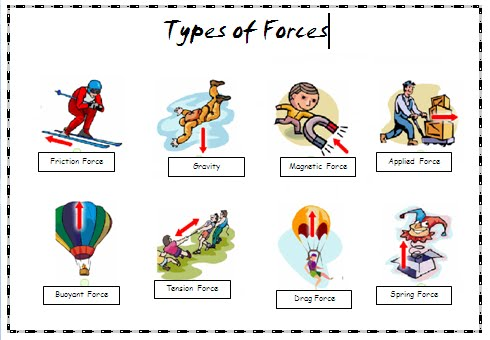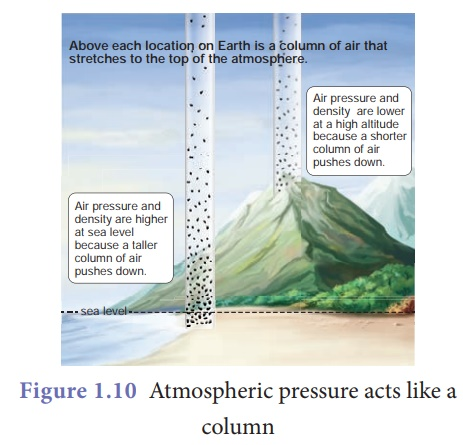# CBSE Class 8 Science Chapter 11 Revision Notes

Chapter 11: Force And Pressure Revision Notes

• Force: A force is a push or a pull that alters or tends to alter an object’s state of rest or uniform motion and its direction or shape.

• The interaction between two objects produces a force.

• Force has both a magnitude (strength of a force) and a direction.Source

• A force applied to an item can cause it to change its state of motion (kicking a football) or shape (squashing a rubber ball).

• A change in an object’s speed, the direction of motion, or both suggests a change in the object’s state of motion (due to a force applied).

• A force can affect an object whether or not it is in touch with it.

TYPES OF FORCES:

There are several different types of forces.

CONTACT FORCES

Forces acting on a body when the source of force is in direct contact with the body are known as contact forces. The point of application of force is where force is applied to an object (or point of contact).

(i) Muscular Force: The force produced by the body’s muscles. To complete our tasks, we rely on the muscular strength of animals such as bullocks, horses, and camels.

(ii) Mechanical Force: The force applied by a machine.

(iii) Frictional Force: This force prevents an object from moving as its direction is always opposite to the direction of motion.

NON-CONTACT FORCES

Forces that act on two bodies without causing physical contact between them.

(i) Magnetic Force: A magnet produces a non-contact force on iron, steel, cobalt, or nickel items.

(ii) Electrostatic Force: The force produced by the attraction of opposite charges or the repulsion of comparable charges.

(ii) Gravitational Forces: The force between any two bodies due to their mass. Gravity is an attractive force.

PRESSURE

Pressure is the force acting on a unit area of a surface.

Pressure = force / area on which it acts

PRESSURE EXERTED BY LIQUIDS AND GASES

• Fluids (liquids and gases) exert pressure just like solids.
• Due to their weight, solids only exert downward pressure, but liquids and gases exert pressure in all directions.
• As a result, liquids and gases exert pressure on the container’s walls.

ATMOSPHERIC PRESSURESource

• The term atmosphere refers to the thick blanket of air that envelopes the world.
• Atmospheric pressure is the pressure applied by the atmosphere.
• Because the fluid pressure inside our bodies counterbalances the atmospheric pressure around us, we are not aware of the enormous atmospheric pressure.
]]>